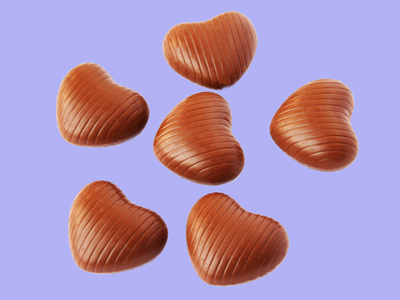4 + 2 gives the same answer as 2 + 4. Whichever way round you put the numbers you still get the answer 6.

# Year 2 Calculation - Addition in a Different Order

This quiz addresses the requirements of the National Curriculum KS1 Maths and Numeracy for children aged 6 and 7 in year 2. Specifically this quiz is aimed at the section dealing with adding in a different order.

In Year 2, children are encouraged to check their addition calculations. They might do this by adding the numbers up again, but in a different order. For example, if they add up 6 + 9 + 4, they may check it by recognising that 6 + 4 makes 10, and 9 more is 19, or they may do it differently by adding 4 + 9 to make 13 then adding 6 to give 19. This is called the commutative property and means that addition calculations can be done in any order to achieve the same answer.

Question 1
What is 10 + 43 + 10 + 2
67
65
75
66
Counting up in tens from 43 and finally adding the 2 makes this calculation easy
Question 2
What is 2 + 14 + 18 + 6?
45
35
50
40
Recognising that there are two pairs of bonds to 20 makes it much easier to solve this
Question 3
What is 16 + 70 + 4?
90
80
78
87
Spotting the bond to 20 makes this calculation become much easier
Question 4
What is 3 + 22?
26
25
27
23
Starting at 3 and counting on 22 would take longer than swapping the order of the numbers around!
Question 5
What is 5 + 5 + 10 + 5 + 5
25
35
30
40
Starting at 10 and counting up in 5s, or adding up all the 5s and then 10 more are both good strategies
Question 6
What is 1 + 1 + 85 + 1 + 1?
89
88
86
98
Starting at 85 and counting on 4 is perfectly acceptable
Question 7
What is 2 + 30 + 8?
37
48
35
40
Adding the 2 and 8 first gives 10, which is easily added to 30
Question 8
What is 12 + 13 + 11 + 10?
54
48
46
44
Adding together all the tens and then the units and combining the two totals is a useful way of solving this
Question 9
What is 1 + 35 + 34?
70
60
75
65
Adding the 1 to the 34 gives 35. 35 + 35 = 70
Question 10
What is 2 + 60 + 4?
75
67
56
66
Adding the smaller numbers first and then adding them to 60 is the best option